# Physics 3 Aug 24 2017 P 3 Challenge

• Slides: 16Physics 3 – Aug 24, 2017 P 3 Challenge – During the annual shuffleboard competition, Renee gives her puck an initial speed of 9. 32 m/s. Once leaving her stick, the puck slows down at a rate of -4. 06 m/s 2. a. Determine the time it takes the puck to slow to a stop. b. Determine the distance which the puck travels before stopping.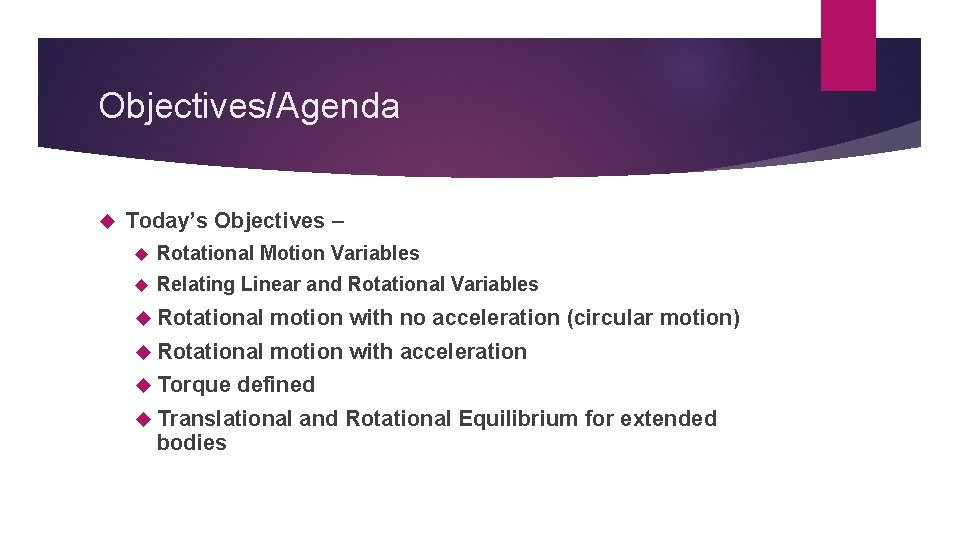Objectives/Agenda Today’s Objectives – Rotational Motion Variables Relating Linear and Rotational Variables Rotational motion with no acceleration (circular motion) Rotational motion with acceleration Torque defined Translational and Rotational Equilibrium for extended bodiesObjectives/Agenda/Assignment Objective: B. 1 Rigid bodies and rotational dynamics (1. 1 – 1. 3) Agenda: Homework Review for p 53, #58 -64, p 60 -61 #11 -12 Assignment: What’s Due? p 16 #1 -6 What’s Next? Read IB B. 1. 4, 1. 5, and 1. 8 p 7 -13 (without rolling)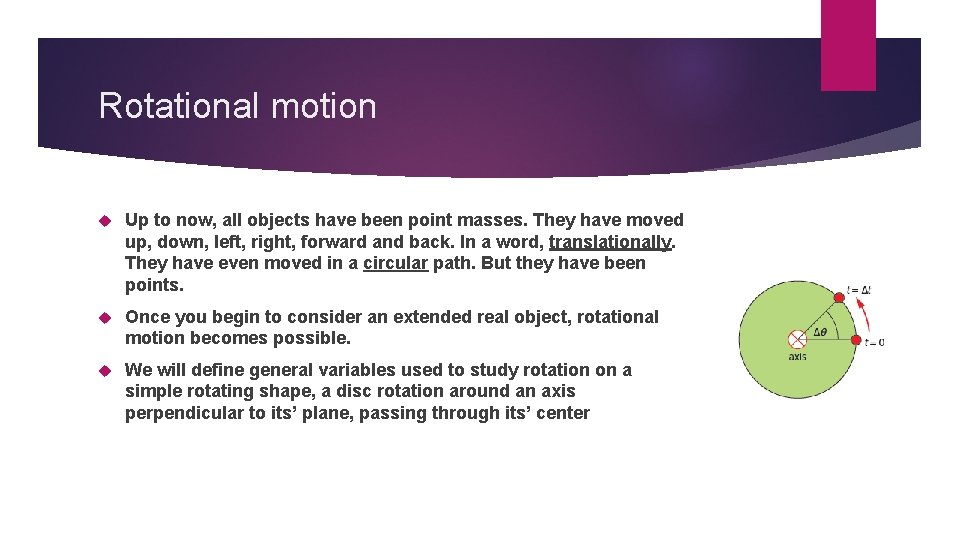Rotational motion Up to now, all objects have been point masses. They have moved up, down, left, right, forward and back. In a word, translationally. They have even moved in a circular path. But they have been points. Once you begin to consider an extended real object, rotational motion becomes possible. We will define general variables used to study rotation on a simple rotating shape, a disc rotation around an axis perpendicular to its’ plane, passing through its’ centerRotational Position and Angular Displacement The reference position for rotation corresponds to a zero angle or positive x axis. The displacement for any rotational position that differs from this position is given the symbol and measured in radians. By convention, counterclockwise rotation represents a positive rotational displacement. Clockwise is negative. But this is only a convention. If a specific problem asks you to use clockwise as positive, that is fine. It’s not wrong. Which way is positive is arbitrary. Why arbitrary? Consider viewing the disc from the other side.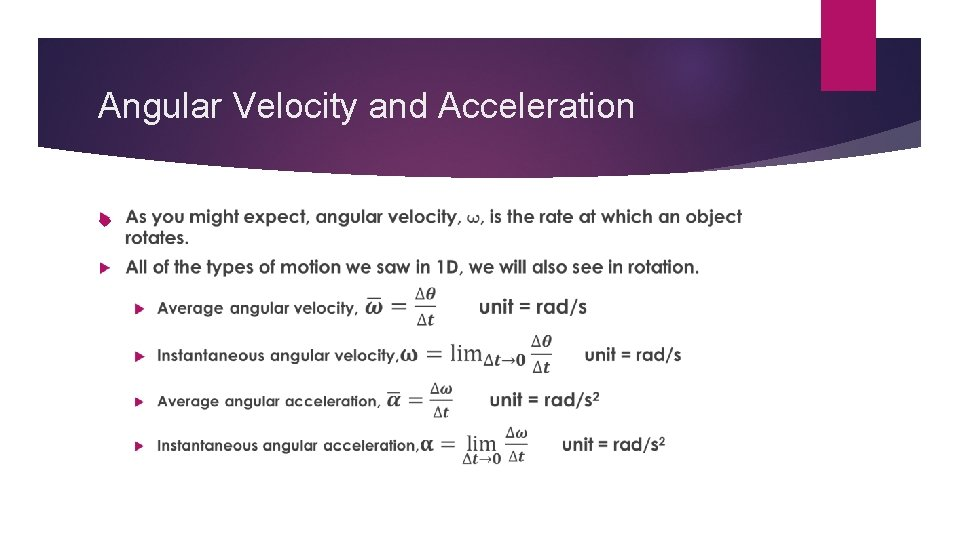Angular Velocity and Acceleration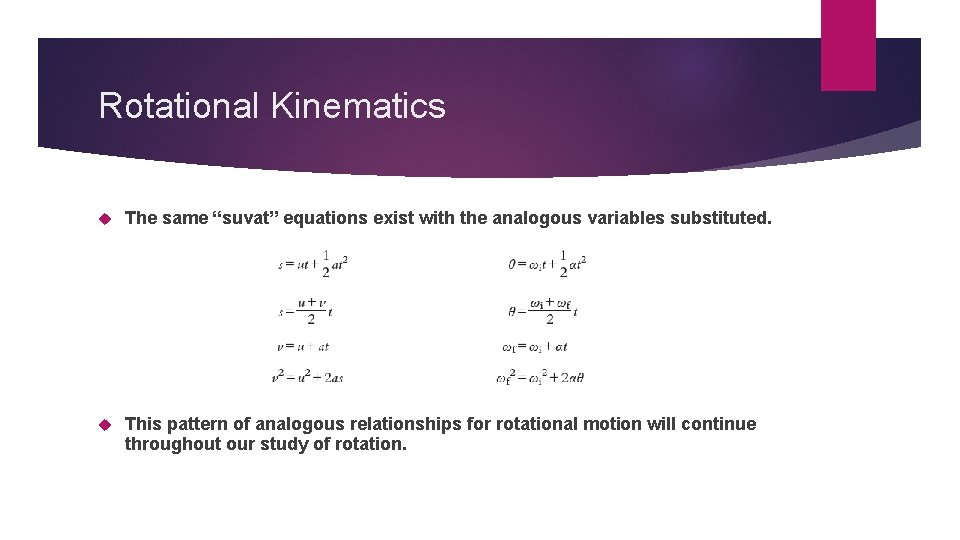Rotational Kinematics The same “suvat” equations exist with the analogous variables substituted. This pattern of analogous relationships for rotational motion will continue throughout our study of rotation.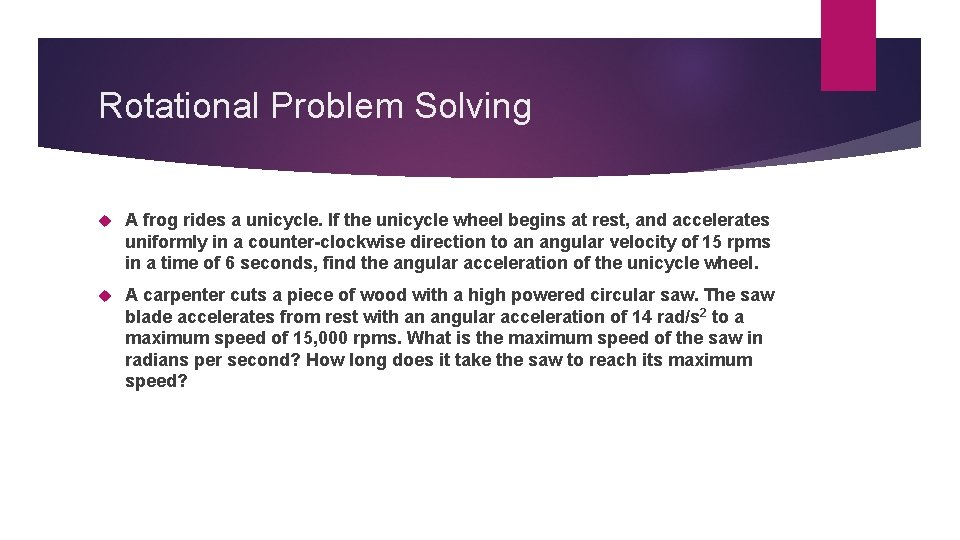Rotational Problem Solving A frog rides a unicycle. If the unicycle wheel begins at rest, and accelerates uniformly in a counter-clockwise direction to an angular velocity of 15 rpms in a time of 6 seconds, find the angular acceleration of the unicycle wheel. A carpenter cuts a piece of wood with a high powered circular saw. The saw blade accelerates from rest with an angular acceleration of 14 rad/s 2 to a maximum speed of 15, 000 rpms. What is the maximum speed of the saw in radians per second? How long does it take the saw to reach its maximum speed?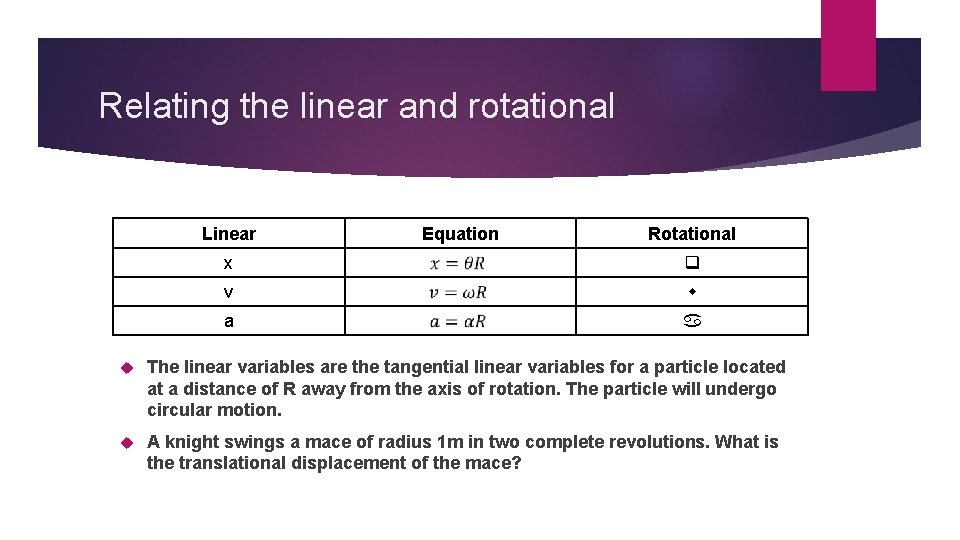Relating the linear and rotational Linear Equation Rotational x v a The linear variables are the tangential linear variables for a particle located at a distance of R away from the axis of rotation. The particle will undergo circular motion. A knight swings a mace of radius 1 m in two complete revolutions. What is the translational displacement of the mace?Torque Torque creates a rotational acceleration. A positive torque is CCW, a negative torque is CW. The maximum torque at a given R is perpendicular to the “lever arm”.Calculating Torque A force of 5. 0 N is applied at the end of a lever that has a length of 2. 0 meters. If the force is applied directly perpendicular to the lever, as shown in the diagram, what is the magnitude of the torque acting the lever?Calculating Torque If the same force as in example 1 is applied at an angle of 30 degrees at the end of the 2. 0 meter lever, what will be the magnitude of the torque?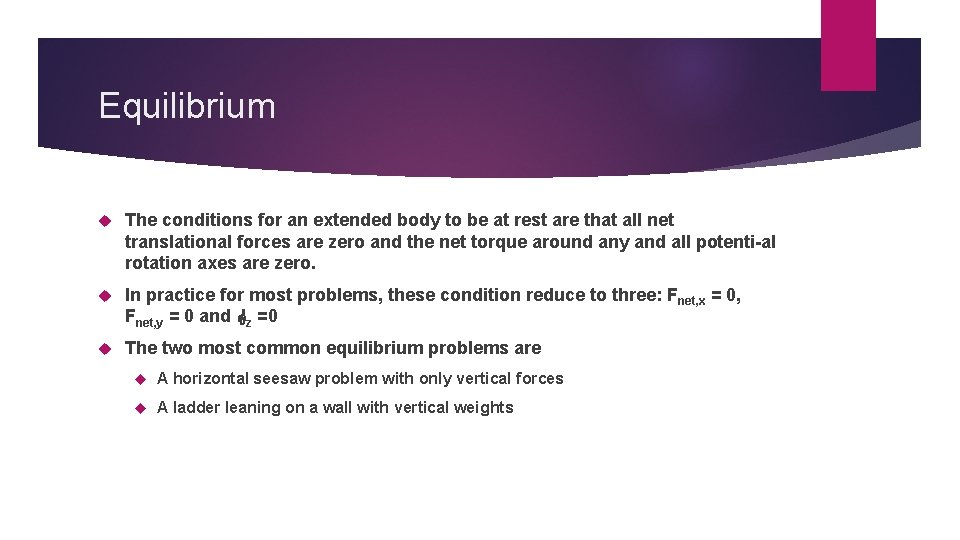Equilibrium The conditions for an extended body to be at rest are that all net translational forces are zero and the net torque around any and all potenti-al rotation axes are zero. In practice for most problems, these condition reduce to three: F net, x = 0, Fnet, y = 0 and z =0 The two most common equilibrium problems are A horizontal seesaw problem with only vertical forces A ladder leaning on a wall with vertical weightsSeesaw problem We want to place another mass on the see-saw of Sample Problem 1 to keep the see-saw from tipping. The only other one we have is a 5. 0 kg mass. Where would we place this to balance the original 3 kg mass that was placed 2. 00 m to the right of the pivot point? What is the normal force at the pivot?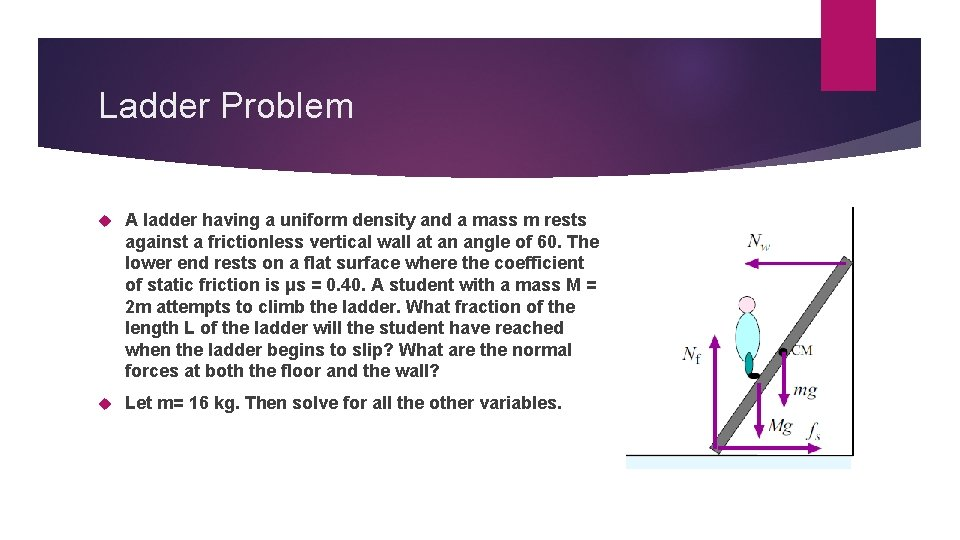Ladder Problem A ladder having a uniform density and a mass m rests against a frictionless vertical wall at an angle of 60. The lower end rests on a flat surface where the coefficient of static friction is μs = 0. 40. A student with a mass M = 2 m attempts to climb the ladder. What fraction of the length L of the ladder will the student have reached when the ladder begins to slip? What are the normal forces at both the floor and the wall? Let m= 16 kg. Then solve for all the other variables.Exit slip and homework Exit Slip – A figure skater is spinning with an angular velocity of +15 rad/s. She then comes to a stop over a brief period of time. During this time, her angular displacement is +5. 1 rad. Determine (a) her average angular acceleration and (b) the time during which she comes to rest. What’s Due? p 16 #1 -6 What’s Next? Read IB B. 1. 4, 1. 5, and 1. 8 p 7 -13 (without rolling)